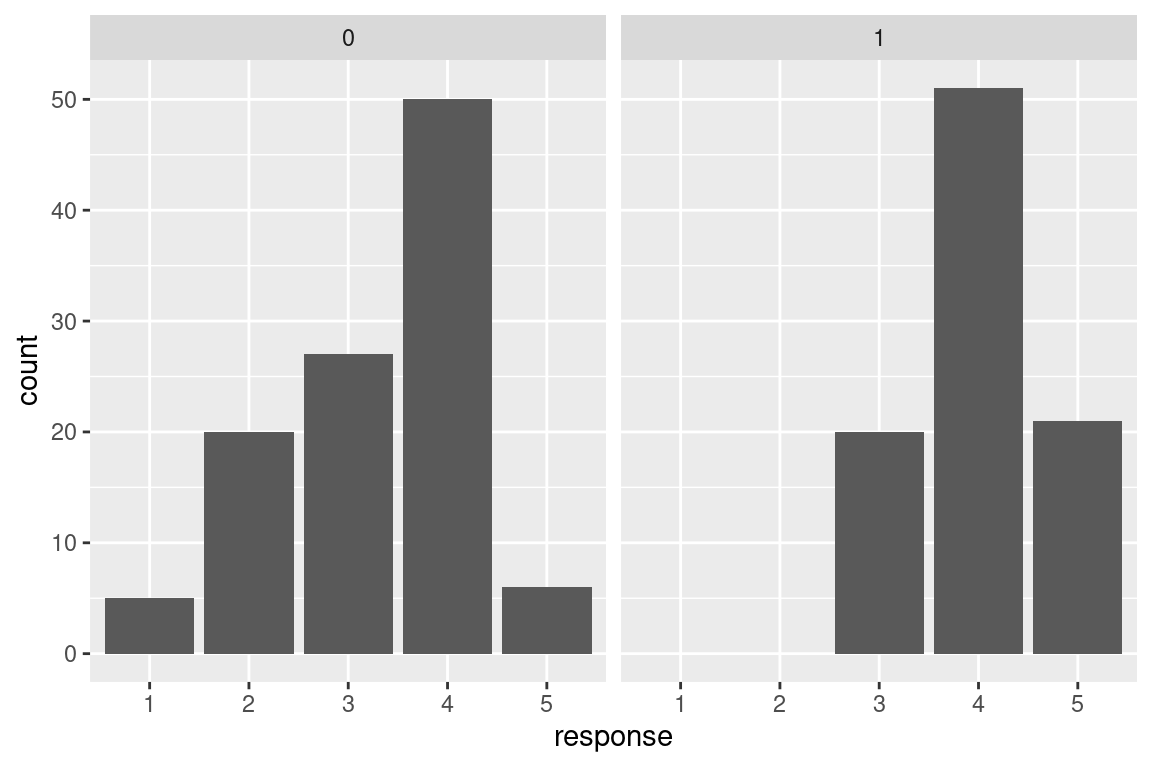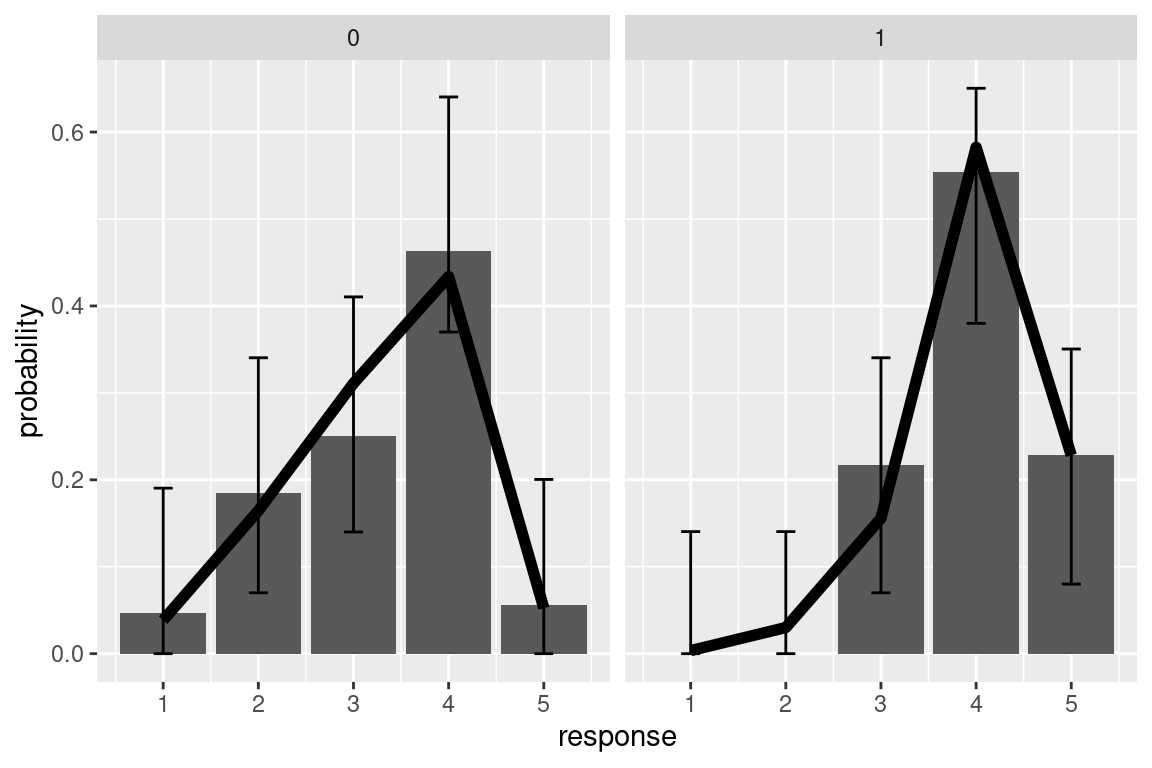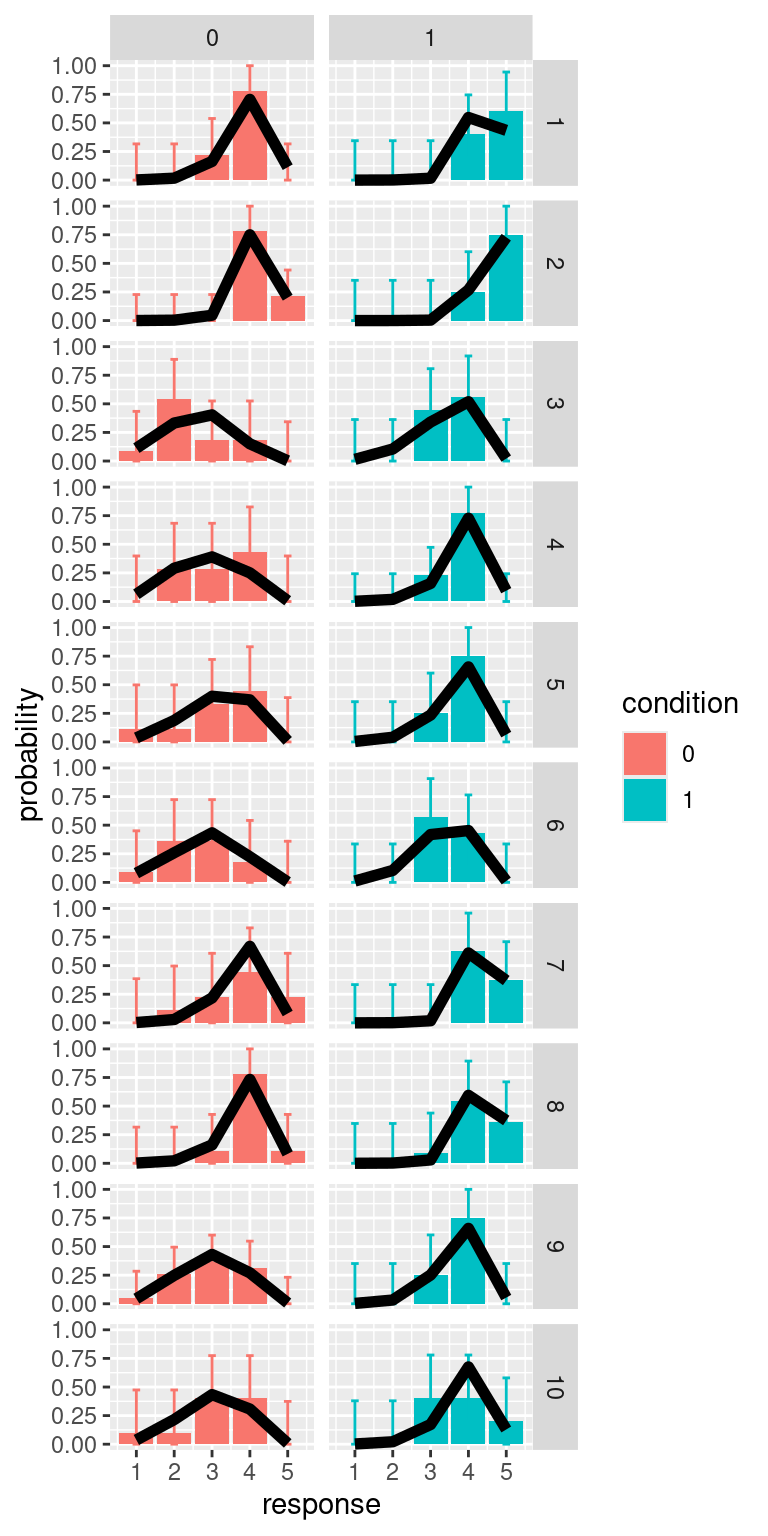# 7 Models for ordinal data

Ordinal-type of variable arise often in psychology. One common example are responses to Likert scales. Although it is very common practice that these are analyzed with a linear model, it is know that this approach can lead to serious inference errors.17 For this reason, the recommended approach is to use a model appropriate for ordinal data. Here I will describe an approach to this, using an ordered logistic regression model (also know as proportional odds model).

## 7.1 Ordered logistic regression

One way to think about this model is by assuming the existence of a continuous latent quantity, call it $$y$$, specified by a logistic probability density function. The latent distribution is partitioned into a series of $$k$$ intervals, where $$k$$ is the number of ordered choice options available to respondents, using $$k+1$$ latent cut-points, $$c_1, \ldots, c_{k+1}$$. By integrating the latent density function within each interval we obtain the ordinal response probabilities $$p_1, \ldots, p_k$$. Other choices are possible (e.g. assuming a normally distributed latent variable would yield an ordered probit model). Beyond mathematical convenience, one advantage of the ordered logit is that coefficient can be interpreted as ordered log-odds, implementing the proportional odds assumption.18

Formally, the model can be notated as

\begin{aligned} p_k & = p\left(c_{k-1} < y \le c_k \mid \mu \right)\\ & = \text{logit}^{-1}\left(c_k - \mu \right) - \text{logit}^{-1}\left(c_{k-1} - \mu \right) \end{aligned}

where $\text{logit}^{-1}(\alpha) = \frac{1}{1+e^{-\alpha}}$ is the cumulative function of the logistic distribution (also known as inverse-logit), and $\mu = \beta_1 x_1 + \ldots + \beta_n x_n$ is the linear part of the model (a linear combination of the $$n$$ predictor variables).

This is the general approach and the formalism used - below I present few examples that illustrates how this work in practice in R.

### 7.1.1 Mixed-effects ordinal regression

R libraries used in this example

library(ggplot2)
library(ordinal)
library(tidyverse)
library(DescTools)

In addition to the above libraries, here I will create a handy R function that gives the probabilities of the categorical responses given a mean value of the latent quantity (indicated with $$\mu$$ above) and a set of cutpoints $$c_1, \ldots, c_{k+1}$$. This will be used both for simulating the data and for plotting the fit of the model.

ordered_logistic <- function(eta, cutpoints){
cutpoints <- c(cutpoints, Inf)
k <- length(cutpoints)
p <- rep(NA, k)
p <- plogis(cutpoints, location=eta, scale=1, lower.tail=TRUE)
for(i in 2:k){
p[i] <- plogis(cutpoints[i], location=eta, scale=1, lower.tail=TRUE) -
plogis(cutpoints[i-1], location=eta, scale=1, lower.tail=TRUE)
}
return(p)
}

For this example we simulate some data. We have two predictors: x1, a continuous predictor that vary with each observation, and d1 a dummy variable that indicate a categorical predictor with 2 levels (e.g. two experimental conditions). The conditions are within-subject, meaningthat each participant (identified by the variable id) is being tested in both conditions.

set.seed(5)
N <- 200
N_id <- 10
dat <- data.frame(
id = factor(sample(1:N_id,N, replace = T)),
d1 = rbinom(N,1,0.5), # dummy variable (0,1) indicate 2 conditions
x1 = runif(n = N, min = 1, max = 10)
)
rfx <- rnorm(length(unique(dat$id)), mean=0, sd=5) LP <- 0.5*dat$x1 + 2*dat$d1 + rfx[dat$id]
for(i in 1:N){
dat$response[i] <- which(rmultinom(1,1, ordered_logistic(LP[i], c(0,2.5, 5,10)))==1) } dat$response <- factor(dat$response) str(dat) #> 'data.frame': 200 obs. of 4 variables: #>$ id      : Factor w/ 10 levels "1","2","3","4",..: 2 9 9 9 5 7 7 3 3 6 ...
#>  $d1 : int 0 0 0 0 0 0 0 1 0 0 ... #>$ x1      : num  6.81 1.49 6.94 3.09 3.97 ...
#>  $response: Factor w/ 5 levels "1","2","3","4",..: 4 1 3 2 2 3 3 4 3 2 ... The dependent variable is categorical with 5 levels - here is a plot of the number of responses per category in the two conditions. We are interested in testing whether the distribution differ across the conditions. ggplot(dat,aes(x=response))+ geom_bar()+ facet_grid(.~d1)We use the clmm() function in the package ordinal to estimate the model. The syntax is similar to what we would use for a linear mixed effect model. Note that in the output the Threshold coefficients are the latent cutpoints $$c_1, \ldots, c_{4}$$ model <- clmm(response ~ x1 + d1 + (1|id), data = dat) summary(model) #> Cumulative Link Mixed Model fitted with the Laplace approximation #> #> formula: response ~ x1 + d1 + (1 | id) #> data: dat #> #> link threshold nobs logLik AIC niter max.grad #> logit flexible 200 -175.75 365.49 291(919) 6.57e-05 #> cond.H #> 8.6e+02 #> #> Random effects: #> Groups Name Variance Std.Dev. #> id (Intercept) 4.224 2.055 #> Number of groups: id 10 #> #> Coefficients: #> Estimate Std. Error z value Pr(>|z|) #> x1 0.55685 0.07516 7.409 1.27e-13 *** #> d1 2.29521 0.36658 6.261 3.82e-10 *** #> --- #> Signif. codes: #> 0 '***' 0.001 '**' 0.01 '*' 0.05 '.' 0.1 ' ' 1 #> #> Threshold coefficients: #> Estimate Std. Error z value #> 1|2 -2.1850 0.8512 -2.567 #> 2|3 0.2853 0.7664 0.372 #> 3|4 2.9453 0.8059 3.655 #> 4|5 7.8126 1.0072 7.757 There is no function that can out-of the box calculate the predictions of the model for us, so this will need some coding. I also use the library DescTools to calculate simultaneous multinomial confidence intervals. In the resulting plot the black line are model fit, and bar the observed responses. # pre-allocate a matrix to store model predictions # note that these are a vector of 5 probabilities for each trial pred_mat <- matrix(NA, nrow=N, ncol=length(unique( dat$response)))

for(i in 1:N){

# first calculate the linear predictor
# by summing all variable as indicated
# in the model formulate, weighted by the coefficients
eta <- dat$x1[i]*model$beta['x1'] +  dat$d1[i]*model$beta['d1'] + model$ranef[dat$id[i]]
# note that + model$ranef[dat$id[i]] adds
# the random intercept for the subjects of observation i

# calculate vector of predicted probabilities
pred_mat[i,] <- ordered_logistic(eta, model$Theta) } # add predictions to dataset pred_dat <- data.frame(pred_mat) colnames(pred_dat) <- paste("resp_",1:ncol(pred_mat),sep="") pred_dat <- cbind(dat, pred_dat) # in order to visalize the predictions, # we first average them for each condition pred_dat %>% pivot_longer(cols=starts_with("resp_"), names_prefix="resp_", values_to = "prob", names_to ="response_category") %>% group_by(d1, response_category) %>% summarise(prob = mean(prob), n=sum(response==response_category)) %>% group_by(d1) %>% mutate(prop_obs = n/sum(n), response=as.numeric(response_category)) -> pred_d1 #> summarise() has grouped output by 'd1'. You can override #> using the .groups argument. # cimpute the multinomial interval pred_d1$CI_lb <- MultinomCI(pred_d1$n)[,"lwr.ci"] *2 pred_d1$CI_ub <- MultinomCI(pred_d1$n)[,"upr.ci"] *2 # note that I multiply for 2 because in the plot each condition # will be in a different panel and the probability will sum to 1 in each panel # visualize (aggregated) ordinal response & prediction # the black line are the predictions of the model ggplot(pred_d1,aes(x=response, y=prop_obs))+ geom_col()+ geom_errorbar(data=pred_d1, aes(ymin=CI_lb, ymax=CI_ub),width=0.2)+ facet_grid(.~d1)+ geom_line(data=pred_d1, aes(y=prob), size=2)+ labs(y="probability") #> Warning: Using size aesthetic for lines was deprecated in ggplot2 #> 3.4.0. #> ℹ Please use linewidth instead. #> This warning is displayed once every 8 hours. #> Call lifecycle::last_lifecycle_warnings() to see where #> this warning was generated.We can repeat the same process also for calculating predictions for individual participants # split by ID pred_dat %>% pivot_longer(cols=starts_with("resp_"), names_prefix="resp_", values_to = "prob", names_to ="response_category") %>% group_by(id, d1, response_category) %>% summarise(prob = mean(prob), n=sum(response==response_category)) %>% group_by(d1, id) %>% mutate(prop_obs = n/sum(n), response=as.numeric(response_category)) -> pred_d1 #> summarise() has grouped output by 'id', 'd1'. You can #> override using the .groups argument. # calculate multinomial CI # we do a loop over all participants and conditions pred_d1$CI_lb <- NA
pred_d1$CI_ub <- NA for(i in unique(pred_d1$id)){
for(cond in c(0,1)){
pred_d1$CI_lb[pred_d1$id==i & pred_d1$d1==cond] <- DescTools::MultinomCI(pred_d1$n[pred_d1$id==i & pred_d1$d1==cond])[,"lwr.ci"]
pred_d1$CI_ub[pred_d1$id==i & pred_d1$d1==cond] <- DescTools::MultinomCI(pred_d1$n[pred_d1$id==i & pred_d1$d1==cond])[,"upr.ci"]
}
}

pred_d1 %>%
mutate(condition = factor(d1)) %>%
ggplot(aes(x=response, y=prop_obs, fill=condition))+
geom_col()+
geom_errorbar(aes(ymin=CI_lb, ymax=CI_ub, color=condition), width=0.2)+
facet_grid(id~d1)+
geom_line(aes(y=prob), size=2)+
labs(y="probability")